"Calculator: The Game" App Analytics: ASO Keyword Monitoring | ASOTools

# "Calculator: The Game" App Analytics: ASO Keyword Monitoring | ASOTools

Are you doing Calculator: The Game app analytics for better google play ASO strategies? Whether Calculator: The Game in google play keep on top? What keyword tactics Calculator: The Game take to rank well in google play? Are app store keywords like "calculator game", "calculator the game", "calculater the game" matter in Calculator: The Game ranking?

Free ASO tools for Google Play & App Store, ASOTools will reveal the app store keywords secrets of Calculator: The Game.

## App Analytics: Calculator: The Game

Calculator: The Game, published by Simple Machine in google play, released on 2017-07-12, and last updated 2019-06-26. It belongs to all category, and all category is ranking no rank in google play. Currently, Calculator: The Game has 35500 ratings, the average rating is 4. Additionally, it had - downloads and - revenue last month in google play worldwide, and the daily active users is -.

More Calculator: The Game info and other app analytics can be found freely in ASOTool.

## ASO Keyword Monitoring: Calculator: The Game

### 1.Calculator: The Game Top 10 App Store Keywords

 Keyword Search Volume KD Related Apps Calculator: The Game's Rank calculator game 9 4605 15 250 1 calculator the game 6 - 3 250 1 calculater the game 2 - 3 250 1 calculator app for gamers 2 - - 250 1 calculator puzle 2 - 11 250 1 caculator the game 2 - 2 250 1 calculator: the game 6 - 3 250 1 calculator puzzle game - - - 250 1 cal game cal cal game - - - 250 1 cal game cal game - - - 250 1

App store keywords matter for ASO. If your app is similar to Calculator: The Game, when you optimize google play keyword ranking, pay attention to the metrics above. ASOTools will show you all of them for free.

Obviously, Calculator: The Game is ranking for "calculator game", "calculator the game", "calculater the game", "calculator app for gamers", "calculator puzle", "caculator the game", "calculator: the game", "calculator puzzle game", "cal game cal cal game", and "cal game cal game" these top 10 keywords in google play.

Also, you'll find Calculator: The Game's position in google play search result. Calculator: The Game is ranking 1 for calculator game, ranking 1 for calculator the game, ranking 1 for calculater the game, and ranking 1 for calculator app for gamers. For more Calculator: The Game keyword monitoring, please visit asotools.io.

### 2. Top 5 Keyword-Related Apps& App Analytics

• calculator game  Related Apps & App Analytics
 Apps Average Star Ratings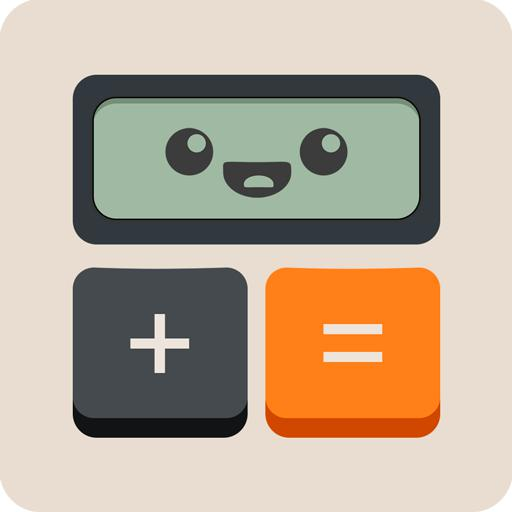Calculator: The Game 4 35500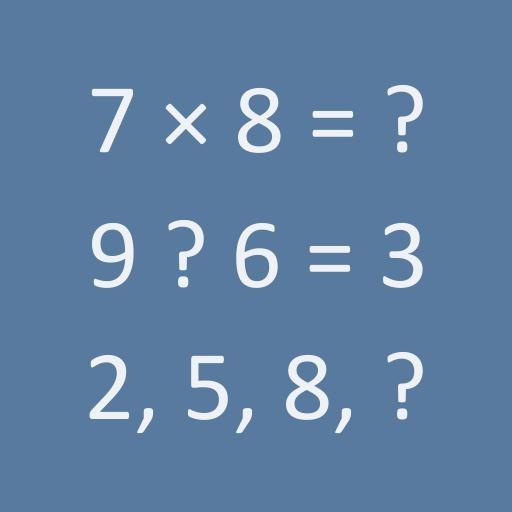Math games - Brain Training 4 25875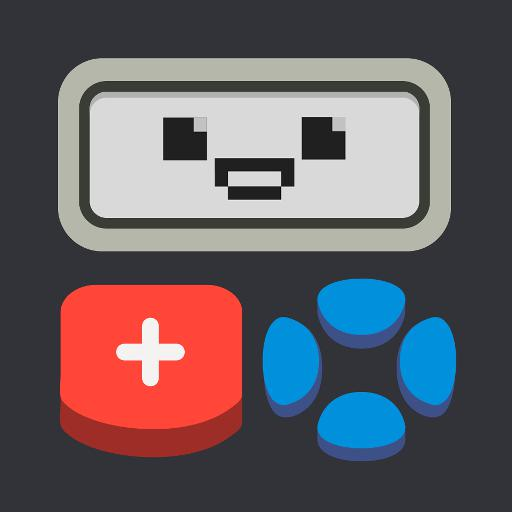Calculator 2: The Game 4 2591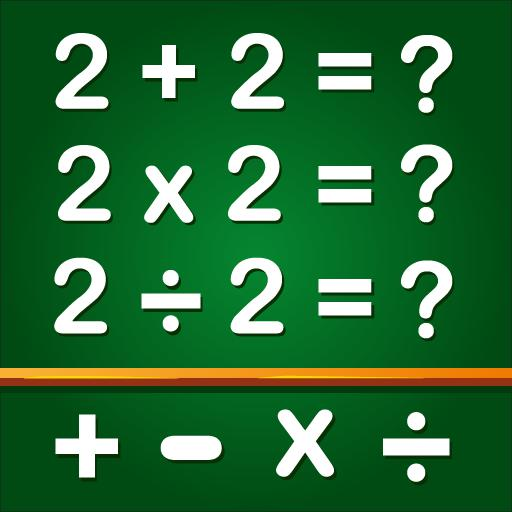Math Games, Learn Add, Subtract, Multiply & Divide 4 194758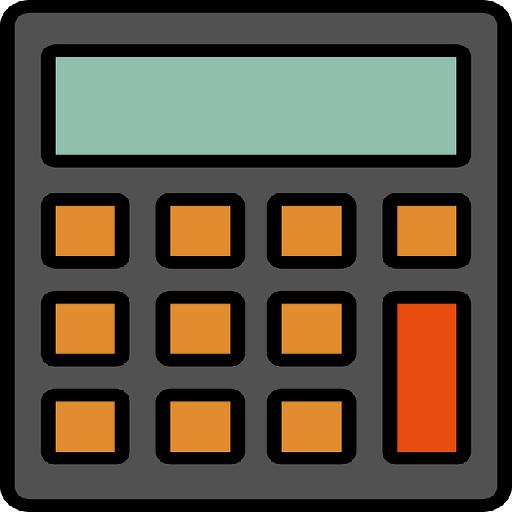Calculator THE GAME 3 6

"calculator game" has 250 apps. Top 5 are Calculator: The Game, Math games - Brain Training, Calculator 2: The Game, Math Games, Learn Add, Subtract, Multiply & Divide, and Calculator THE GAME.

• calculator the game  Related Apps & App Analytics
 Apps Average Star RatingsCalculator: The Game 4 35500Calculator 2: The Game 4 2591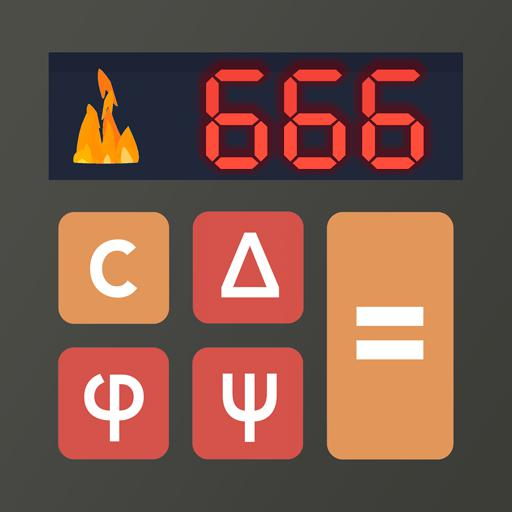The Devil's Calculator: A Math Puzzle Game 4 569Calculator THE GAME 3 6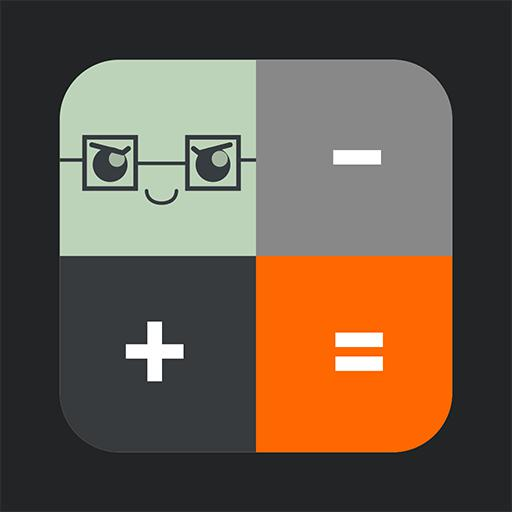Calculator Puzzle: Fun Math Game 3 44

"calculator the game" has 250 related apps in total. Top 5 are Calculator: The Game, Calculator 2: The Game, The Devil's Calculator: A Math Puzzle Game, Calculator THE GAME, and Calculator Puzzle: Fun Math Game.

• calculater the game  Related Apps & App Analytics
 Apps Average Star RatingsCalculator: The Game 4 35500Calculator 2: The Game 4 2591Calculator Puzzle: Fun Math Game 3 44Calculator THE GAME 3 6The Devil's Calculator: A Math Puzzle Game 4 569

"calculater the game" has 250 related apps in google play. Top 5 are Calculator: The Game, Calculator 2: The Game, Calculator Puzzle: Fun Math Game, Calculator THE GAME, and The Devil's Calculator: A Math Puzzle Game.

• calculator app for gamers  Related Apps & App Analytics
 Apps Average Star RatingsCalculator: The Game 4 35500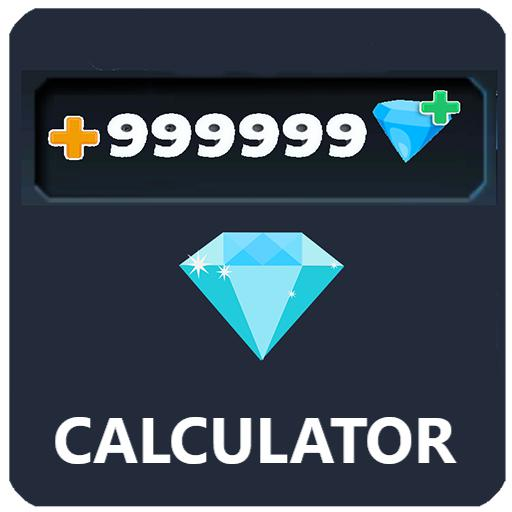Diamonds Calculator for Gamers ML - -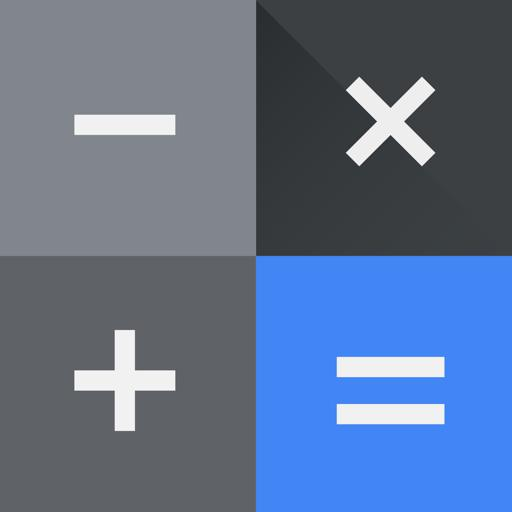Calculator 4 337686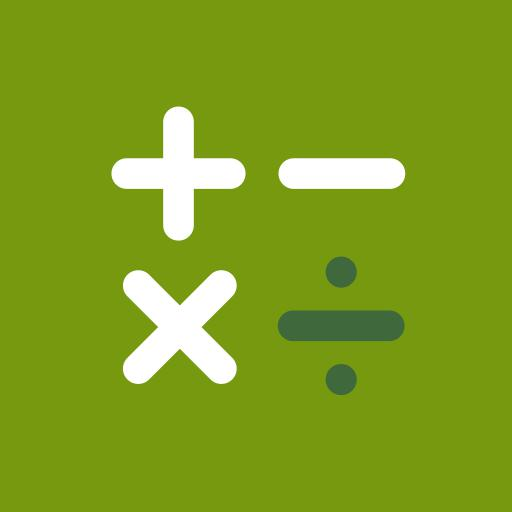Samsung Calculator - -Calculator 2: The Game 4 2591

"calculator app for gamers" has 250 related apps. Top 5 apps are Calculator: The Game, Diamonds Calculator for Gamers ML, Calculator, Samsung Calculator, and Calculator 2: The Game.

• calculator puzle  Related Apps & App Analytics
 Apps Average Star RatingsCalculator: The Game 4 35500The Devil's Calculator: A Math Puzzle Game 4 569Calculator 2: The Game 4 2591Calculator Puzzle: Fun Math Game 3 44Calculator 4 337686

250 apps are ranking for "calculator puzle" in google play. Top 5 apps are Calculator: The Game, The Devil's Calculator: A Math Puzzle Game, Calculator 2: The Game, Calculator Puzzle: Fun Math Game, and Calculator.

As you can see, Calculator: The Game, Calculator: The Game, and Math games - Brain Training are ranking for calculator game, also, Calculator: The Game, Calculator: The Game, Calculator 2: The Game and Calculator THE GAME are all competing the keyword calculator the game.

Before promoting your apps in google play, you'd better perform app analytics for better ASO tactics, and ASOTools, free ASO tools will help you with App store keywords optimization.